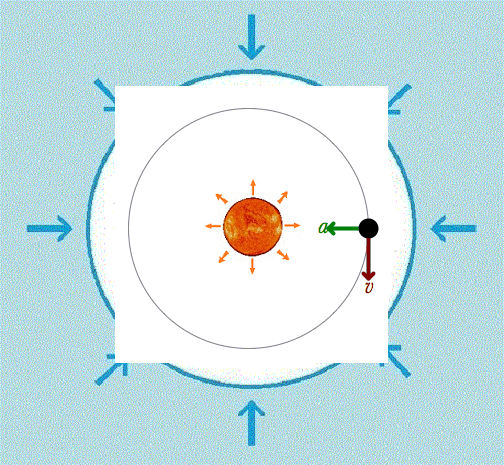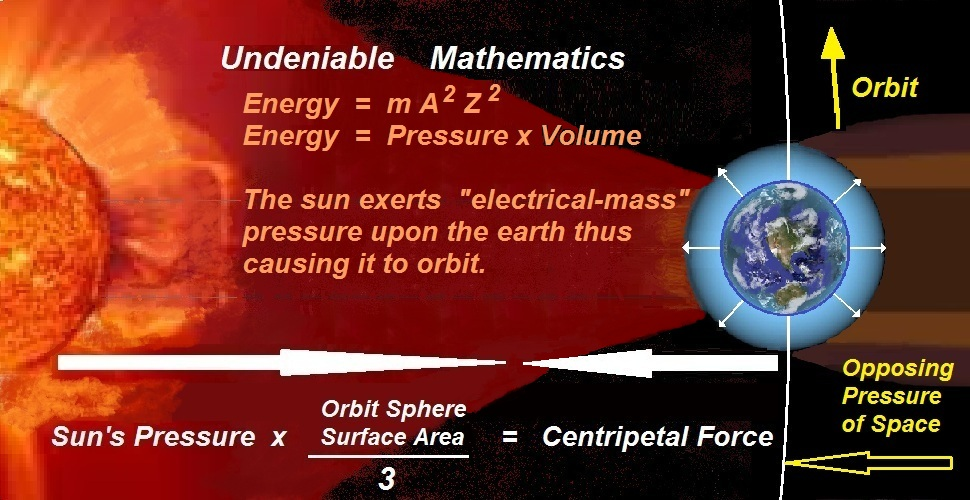E i n s t e i n G r a v i t y . c o m

Monumental   Scientific   Discovery  !

The  Truth  Regarding  The  Energy  Equation

First off we need to study this incredible video, a true gem.

It now has over a million views for a very very good reason  !

Mathematical   Pi   Certainly   Needs   Correction.

Energy  =  mass x Velocity

Energy  =  m x AZ

m/AZ  =  q  =  electrical-mass

Energy  =  q x AZ x AZ

Energy  =  q(AZ)2

Energy  =  qA2Z2From the image above we can plainly see that it is  Light x Heat  (energy) that is coming off of the sun that is driving the orbit of the earth.  Each of the different equations below are all equal to that same one energy equation which is ( Mass x Velocity ) or ( Electrical-mass x Velocity2 )  or  (a planet orbiting).

But you might say  "Hey, energy is not equal to Mass x Velocity it's equal to Mass x Velocity squared".

And without hesitation pure physics would boldly reply; "O.k. hold tight, let's go slow and methodical".  Because just like the Pi video that you just saw, the historic equation for energy has also been grossly mistaken.

In the table below (AZ = Temp = Velocity) and whether it's;

1.  Light x Distance
2.  Force x Volume / Area x Velocity
3.  Pressure / Temperature x Volume    or
4.  Mass x Velocity

They are all exactly equal.     Equal to a planet orbiting.

 1 2 3 4
 Light  x  Distance = Force  x  Volume  /  Area  x  Velocity = Pressure / AZ  x  Volume = Mass x Velocity m/Z x AZ2 mA  x  A3Z6 / A2Z4 x AZ m/AZ4 / AZ  x  A3Z6 mAZ

Now when we multiply the long hidden factors of  "Velocity"  into each of the  4 equations they then turn into the energy values that are much more familiar to us;

 1 2 3 4
 Light  x  Heat = Force  x  Distance = Pressure  x  Volume = Mass x Velocity2

But just because the above equations all posses an extra velocity factor doesn't mean that the extra factor is surely supposed to be there.  And this all stems back to 1730 a.d. when Willem Gravesande was dropping a lead ball into a block of soft clay when he noticed that the Velocity was squared proportional to the energy dent within the clay.

We can see that what he didn't realize was that his use of Velocity squared was exactly parallel to the Pi video that you just saw.  Willem Gravesande did not realize that his Velocity2 factor was pertaining to both the  "motion"  of the falling ball  - as well as -  the  "atomic composition"  of the ball itself.

The reason that all of the above equations truly equal "mAZ", in the same way that Pi should truly be seen as 1/2 TAU, is because Einstein's "m" is not ordinary mass, it is Mass divided by Temperature (m/AZ). See this page for a full explanation - GRAVESANDE.

 1 2 3 4
 Light  x  Heat (wrongly contains an extra hidden factor of AZ) = Force  x  Distance (wrongly contains an extra hidden factor of AZ) = Pressure  x  Volume (wrongly contains an extra hidden factor of AZ) = Mass x Velocity2 (wrongly contains an extra hidden factor of AZ)

When we compare the above table to the image below we can plainly see how ALL energy equations, beginning with # 4 (Gravesande's falling ball) all the way left to the very origin of all energy (the sun) each equation possesses a hidden value of Velocity that does not truly belong as part of the equation.And this is most evident with the equation  # 2  in the table.  For when we highlight that equation we see that it is also equal to;

Force  x  Distance2  /  Heat

mA  x  A2Z4  /  A2Z3

mAZ

Force is originating from the sun in the form of  "Mass x Acceleration" (mass combusting) (waves of accelerated electrical-mass) and as it travels a Distance it then hits earth which then causes the earth to orbit a Distance.  So the parallels of mass in the equation are (the electrical-mass coming off of the sun) and (the mass of planet earth in space).  They both then travel a Distance.  And the two actions are engaged in the conservation of momentum that is separated by the Heat content of that portion of space.

True Energy  =  mass x Velocity.

m x AZ

True Energy  =  Force occuring for a period of Time.

mA x Z

True Energy  =  Electrical-mass x Velocity x Velocity.

q x AZ x AZ   =   mAZ

Willem Gravesande's experimental lead ball being Accelerated by Gravity for a period of Time.

And at the end of that period of Time, then fully capable of doing work, expending energy.

Give this news to someone today  !